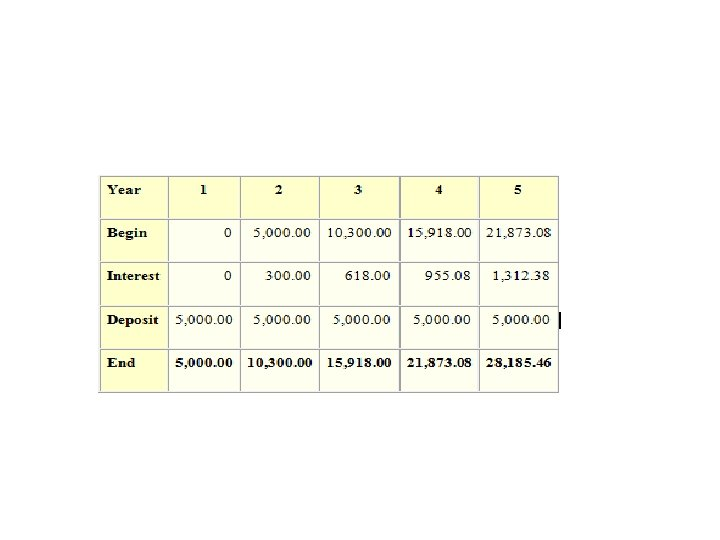Скачать презентацию Time Value of Money Why TIME

c050c5da1cf9958ab0322566c90943d5.ppt

• Количество слайдов: 17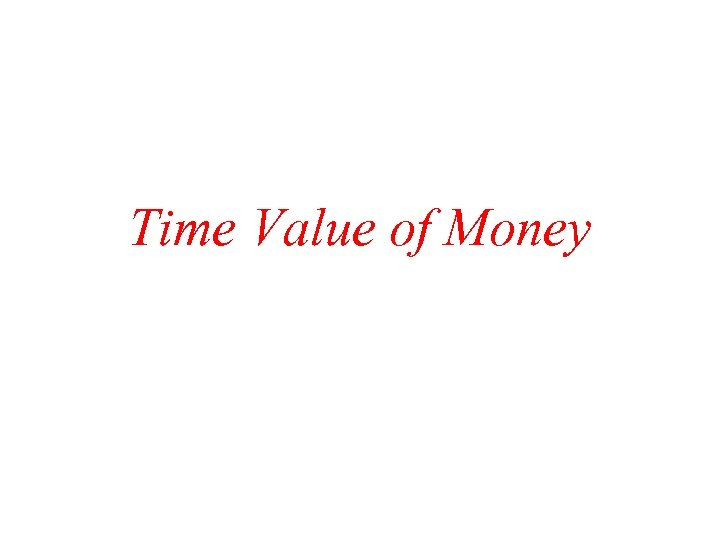Time Value of Money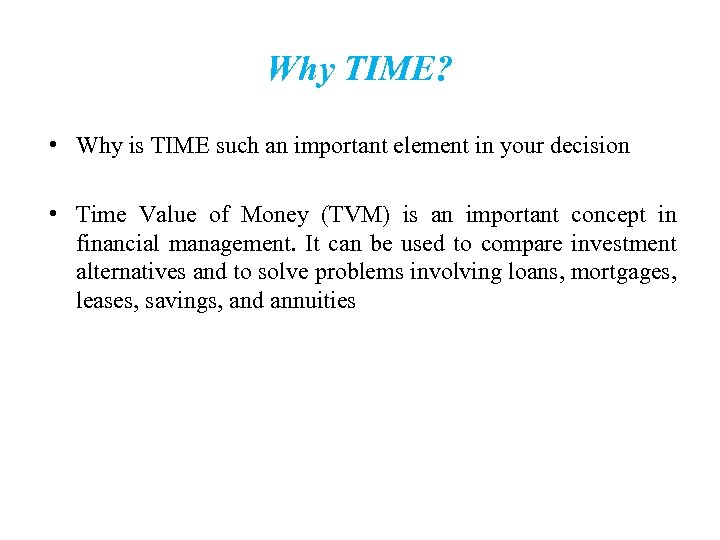Why TIME? • Why is TIME such an important element in your decision TIME • Time Value of Money (TVM) is an important concept in financial management. It can be used to compare investment alternatives and to solve problems involving loans, mortgages, leases, savings, and annuities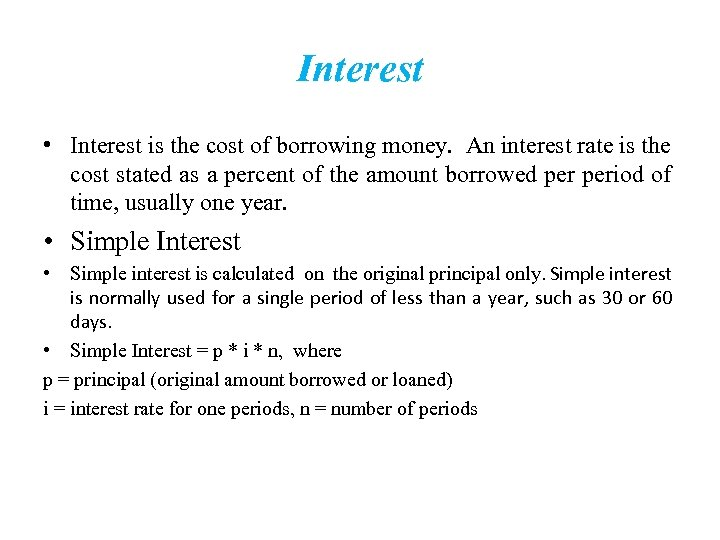Interest • Interest is the cost of borrowing money. An interest rate is the cost stated as a percent of the amount borrowed period of time, usually one year. • Simple Interest • Simple interest is calculated on the original principal only. Simple interest is normally used for a single period of less than a year, such as 30 or 60 days. • Simple Interest = p * i * n, where p = principal (original amount borrowed or loaned) i = interest rate for one periods, n = number of periods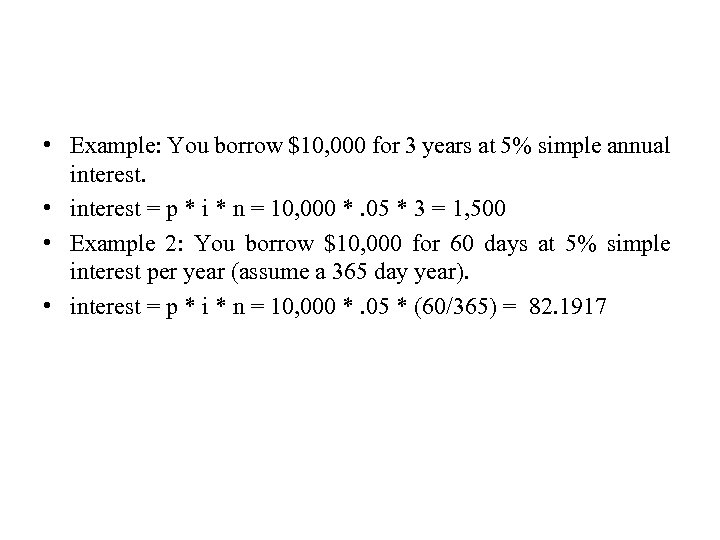• Example: You borrow \$10, 000 for 3 years at 5% simple annual interest. • interest = p * i * n = 10, 000 *. 05 * 3 = 1, 500 • Example 2: You borrow \$10, 000 for 60 days at 5% simple interest per year (assume a 365 day year). • interest = p * i * n = 10, 000 *. 05 * (60/365) = 82. 1917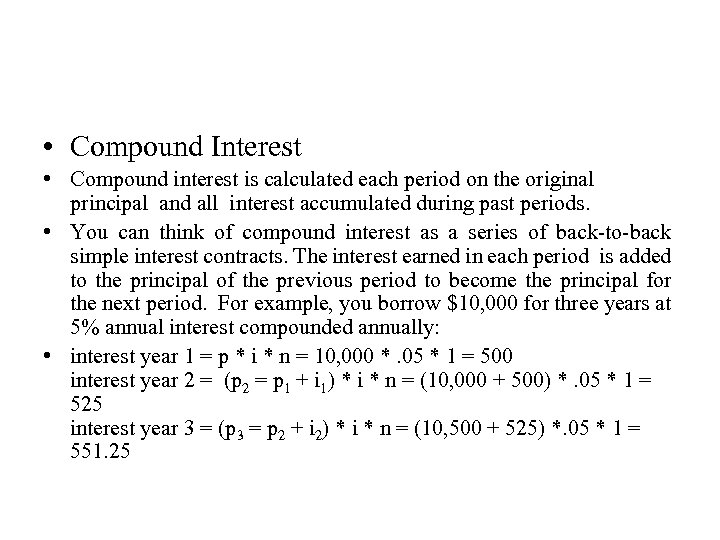• Compound Interest • Compound interest is calculated each period on the original principal and all interest accumulated during past periods. • You can think of compound interest as a series of back-to-back simple interest contracts. The interest earned in each period is added to the principal of the previous period to become the principal for the next period. For example, you borrow \$10, 000 for three years at 5% annual interest compounded annually: • interest year 1 = p * i * n = 10, 000 *. 05 * 1 = 500 interest year 2 = (p 2 = p 1 + i 1) * i * n = (10, 000 + 500) *. 05 * 1 = 525 interest year 3 = (p 3 = p 2 + i 2) * i * n = (10, 500 + 525) *. 05 * 1 = 551. 25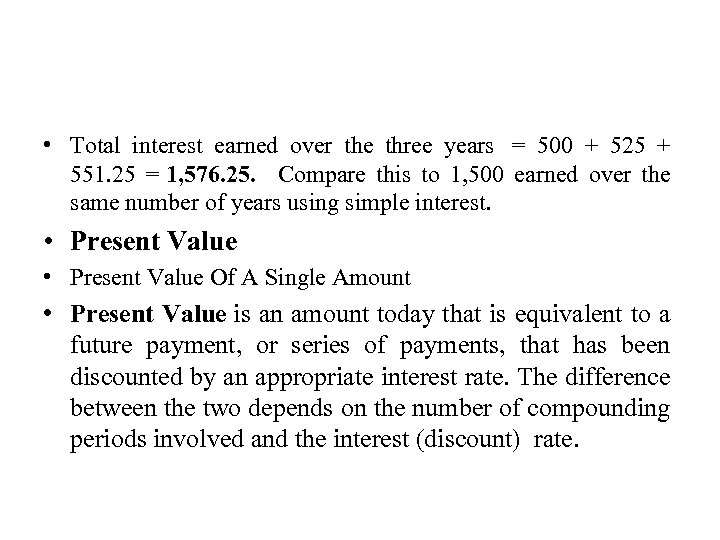• Total interest earned over the three years = 500 + 525 + 551. 25 = 1, 576. 25. Compare this to 1, 500 earned over the same number of years using simple interest. • Present Value Of A Single Amount • Present Value is an amount today that is equivalent to a future payment, or series of payments, that has been discounted by an appropriate interest rate. The difference between the two depends on the number of compounding periods involved and the interest (discount) rate.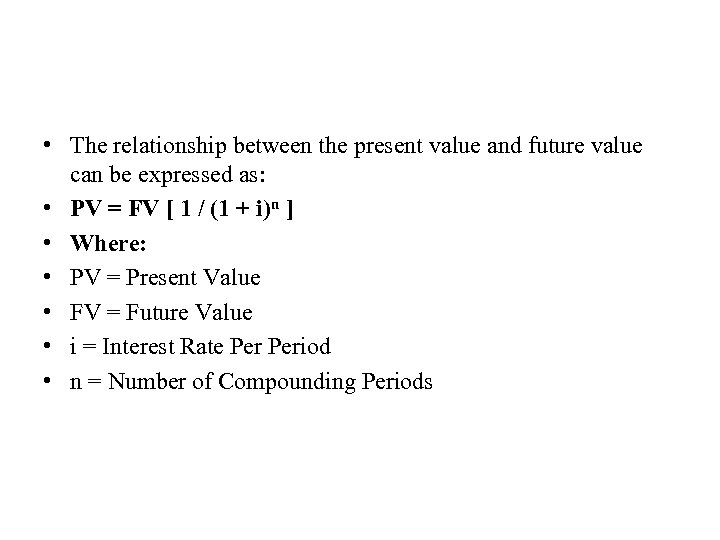• The relationship between the present value and future value can be expressed as: • PV = FV [ 1 / (1 + i)n ] • Where: • PV = Present Value • FV = Future Value • i = Interest Rate Period • n = Number of Compounding Periods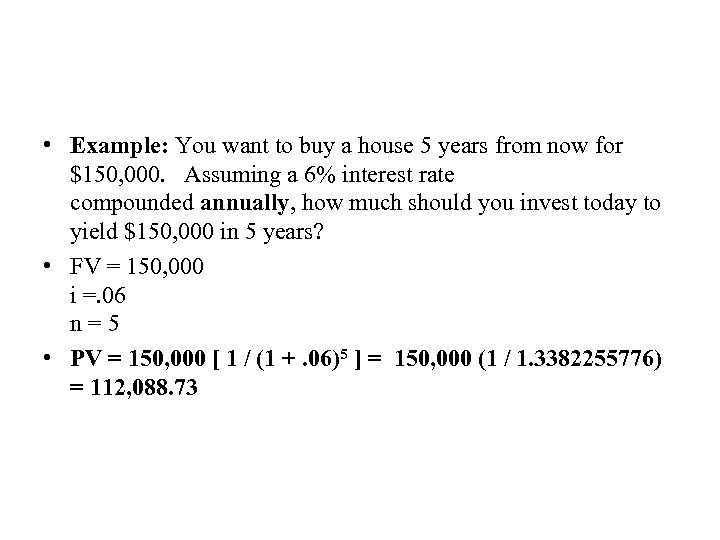• Example: You want to buy a house 5 years from now for \$150, 000. Assuming a 6% interest rate compounded annually, how much should you invest today to yield \$150, 000 in 5 years? • FV = 150, 000 i =. 06 n = 5 • PV = 150, 000 [ 1 / (1 +. 06)5 ] = 150, 000 (1 / 1. 3382255776) = 112, 088. 73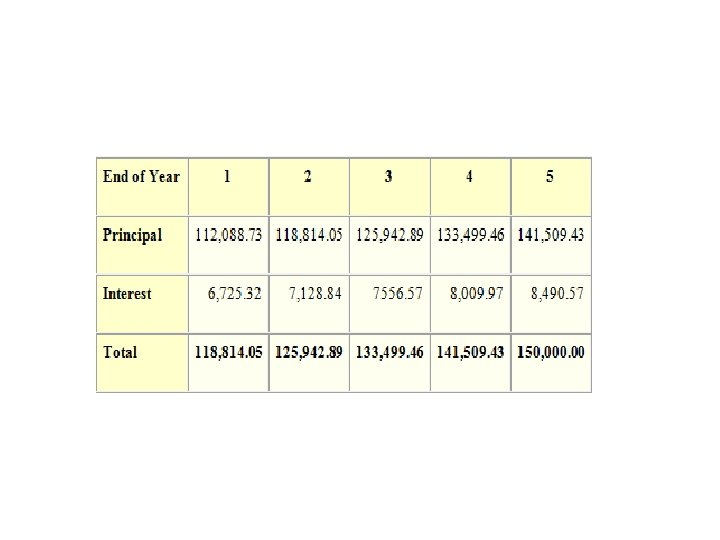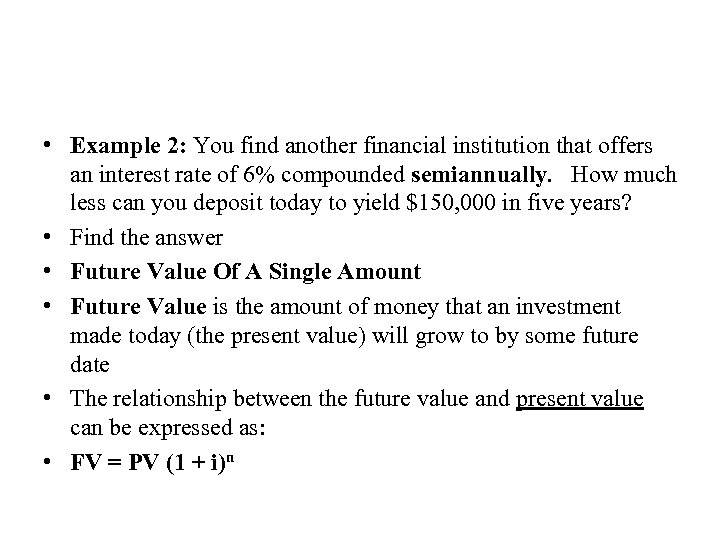• Example 2: You find another financial institution that offers an interest rate of 6% compounded semiannually. How much less can you deposit today to yield \$150, 000 in five years? • Find the answer • Future Value Of A Single Amount • Future Value is the amount of money that an investment made today (the present value) will grow to by some future date • The relationship between the future value and present value can be expressed as: • FV = PV (1 + i)n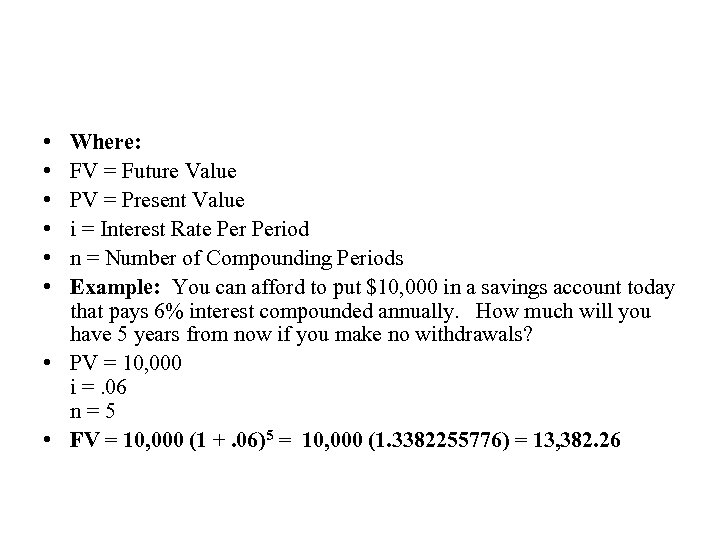• • • Where: FV = Future Value PV = Present Value i = Interest Rate Period n = Number of Compounding Periods Example: You can afford to put \$10, 000 in a savings account today that pays 6% interest compounded annually. How much will you have 5 years from now if you make no withdrawals? • PV = 10, 000 i =. 06 n = 5 • FV = 10, 000 (1 +. 06)5 = 10, 000 (1. 3382255776) = 13, 382. 26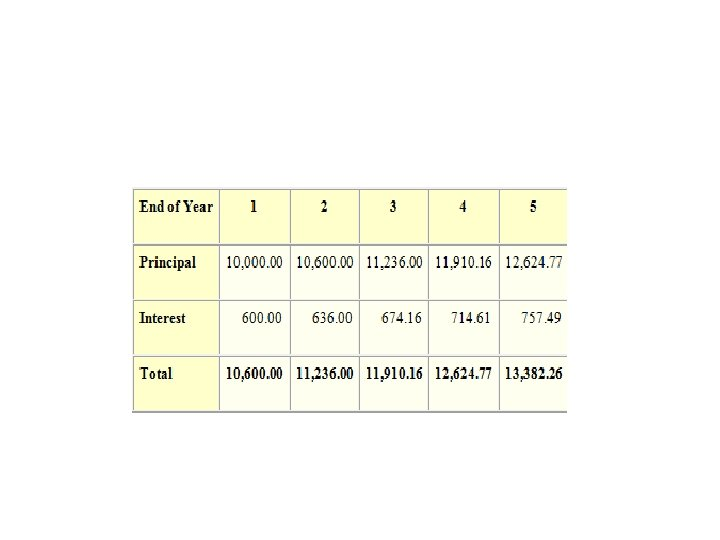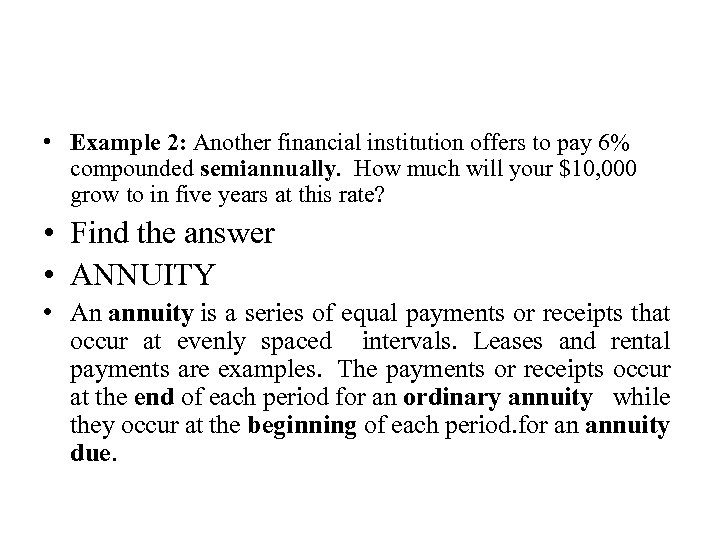• Example 2: Another financial institution offers to pay 6% compounded semiannually. How much will your \$10, 000 grow to in five years at this rate? • Find the answer • ANNUITY • An annuity is a series of equal payments or receipts that occur at evenly spaced intervals. Leases and rental payments are examples. The payments or receipts occur at the end of each period for an ordinary annuity while they occur at the beginning of each period. for an annuity due.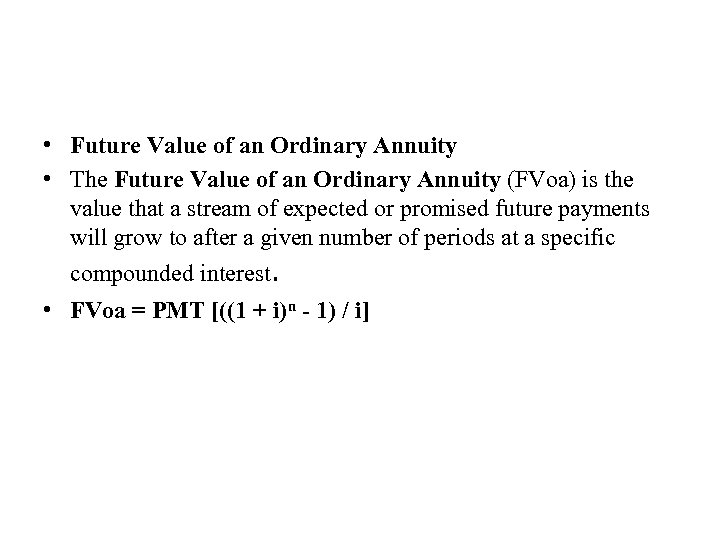• Future Value of an Ordinary Annuity • The Future Value of an Ordinary Annuity (FVoa) is the value that a stream of expected or promised future payments will grow to after a given number of periods at a specific compounded interest. • FVoa = PMT [((1 + i)n - 1) / i]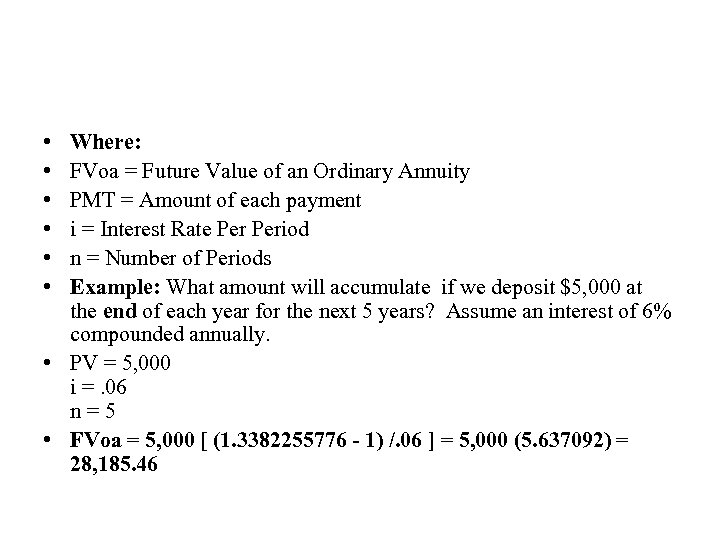• • • Where: FVoa = Future Value of an Ordinary Annuity PMT = Amount of each payment i = Interest Rate Period n = Number of Periods Example: What amount will accumulate if we deposit \$5, 000 at the end of each year for the next 5 years? Assume an interest of 6% compounded annually. • PV = 5, 000 i =. 06 n = 5 • FVoa = 5, 000 [ (1. 3382255776 - 1) /. 06 ] = 5, 000 (5. 637092) = 28, 185. 46